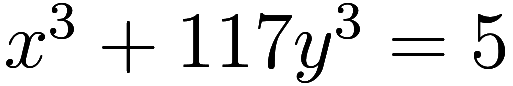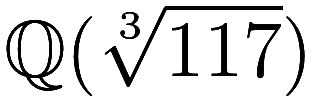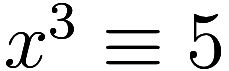2019 February 24
originally posted on facebook

In 1971 the proof below was given thathas no integer solutions, using some difficult computations in the field.

In 1973 it was observed that the equation reduces tomod 9, which has no solutions.

https://www.cambridge.org/core/services/aop-cambridge-core/content/view/BE6D6D5BF821E25BB03D93AE3A13C19F/S0008439500057970a.pdf/on_d_j_lewiss_equation_x_3117y_3_5.pdf

Finkelstein, R., & London, H. (1971). On D. J. Lewis’s Equation x^3 + 117y^3 = 5. Canadian Mathematical Bulletin, 14(1), 111-111. doi:10.4153/CMB-1971-020-x